# Artificial neural network modeling of hydrogen sulphide gas coolers ensuring extrapolation capability

Artificial neural network modeling of hydrogen sulphide gas coolers ensuring extrapolation capability

Andres A. Sánchez-EscalonaEver Góngora-Leyva

Engineering Division, Moa Nickel S.A., Moa 83-310, Holguín, Cuba

Faculty of Metallurgy and Electromechanical, Metallurgical Mining Higher Institute, Moa 83-330, Holguín, Cuba

Corresponding Author Email:
aescalon@moanickel.com.cu
Page:
348-356
|
DOI:
https://doi.org/10.18280/mmep.050411
31 August 2018
|
Accepted:
4 December 2018
|
Published:
31 September 2018
| Citation

OPEN ACCESS

Abstract:

Hydrogen sulphide coolers are jacketed shell-and-tube heat exchangers designed to cool down the gas from 416.15 K to 310.15 K, as well as to remove sulphur carryovers. It is difficult to accurately compute their performance by traditional methods, since thermal analysis is based on several simplifications and empirical correlations. To overcome this limitation, the aim of present research was to propose an artificial neural network model for prediction of coolers outputs, using the mean absolute percentage error, correlation coefficient and extrapolation capability as selection criteria. Structure optimization was carried out through a network growing strategy, using 120 experimental data points for networks training, validation and testing. Model generalization was verified by comparing responses against the predictions of a validated phenomenological model, based on the ε-NTU method, for one set of 20 unseen data points. Best performance was obtained with the 6-5-4-3 multilayer perceptron, using the Levenberg-Marquardt learning algorithm. 99.47 % overall correlation and 0.33 % mean absolute percentage error were achieved when computing the hydrogen sulphide and water streams outlet temperatures. Despite the high prediction performance, a few model responses were found deprived of physical sense.

Keywords:

artificial neural network, extrapolation, heat exchanger, hydrogen sulphide, modeling

1. Introduction

Hydrogen sulfide (H2S) is a toxic and flammable gas that occurs both naturally and from human-made processes. Although it is usually obtained from “sour gas” contained in natural gas, the commercial way to produce a high-purity stream is by reacting hydrogen with molten sulphur at 698 - 753 K and 800 kPa. Applications for this compound include nuclear industry heavy water, sulfur and sulfuric acid production, nickel plus cobalt hydro metallurgical extraction, methyl mercaptan and gas odorizers manufacture, among others .

Present research was carried out in an online system of hydrogen sulphide gas coolers, belonging to a 99.8%-purity production plant. The function of these jacketed shell-and-tube heat exchangers is to cool down the main process stream from 416.15 K to 310.15 K, and remove the remaining sulphur carried over by the gas. Because of the complex nature of the heat transfer processes simultaneously occurring within mentioned equipment, their performance has not been accurately calculated at the plant site by traditional rating methods. A set of simplifications and assumptions were introduced by the engineers to make the theoretical model simple enough for the analysis [2-3]. In this respect, novel techniques are required for improved prediction of the heat exchangers outputs.

Artificial neural networks (ANN) have been successfully employed for various heat transfer applications and thermal analysis of heat exchangers. Once trained, they are a quick and reliable tool for anticipating thermal processes performance. Other advantages are precise approximations of complex non-linear problems, greater efficiency than phenomenological models and “black box” approach, hence not requiring a detailed knowledge of the physical phenomena describing the system under analysis [3-6].

Several authors have used ANN for shell-and-tube heat exchangers modeling. Pandharipande et al.  optimized a multilayer feed forward network, also called a perceptron, in order to estimate hot and cold water outlet temperatures as a function of flow rates and inlet temperatures. The research was carried out at lab scale, using a 0.39 m2 heat exchanger. Mandavgane & Pandharipande  conducted a similar research, employing the same experimental setup for two study cases: water–20% glycerin and water–40% glycerin. A more rigorous study was implemented by Xie et al. , who investigated three shellside heat exchanger configurations. Prediction of outlet temperature differences for each fluid and overall heat transfer rates was performed, by using eight independent variables: Inlet temperatures, tubeside and shellside Reynolds number, tubes quantity, center tube diameter, baffles number and baffle pitch.

Another model was further developed by Moghadassi et al. , establishing 22 networks for anticipating different fluids properties and equipment parameters. Data sets were collected form Kern’s book, TEMA standard and Perry’s handbook. Different from above-mentioned studies, an industrial scale research was carried out by Jasim  for prediction of water and air exit temperatures in an online air cooler. Selected input variables were water and air inlet temperatures, as well as air mass flowrate. Network training and testing were accomplished by means of a 200 experimental data points.

Lastly, Iyengar  performed thermal analysis of a one-shell-pass two-tube-passes heat exchanger, with design parameters taken from a literature case study. Kern’s method correlations were used to generate 1080 data points. Independent variables were velocity, baffle distance, pipe thickness and tube pitch, while outputs consisted of overall heat transfer coefficient and pressure drops.

On previous researches, statistical parameters like mean absolute percentage error (MAPE), mean squared error (MSE), coefficient of determination (${{R}^{2}}$) and correlation coefficient (R) were used to choose the network structure that performs better. However, generalization ability was not evaluated even when overfitting and extrapolation errors are well-known limitations of ANN [4, 6]. Industry equipment simulations require not only to be accurate, but also reasonably effective beyond experimental data. Taking these facts into account, the objective of present study is to propose an ANN model for prediction of hydrogen sulphide gas coolers outputs, assessing the extrapolation capability during the network structure optimization process.

2. System Description and Experimental Setup

The studied system consists of four jacketed shell-and-tube heat exchangers, having “BEU with external jacket” type designation according to the TEMA standard . They are three-fluid heat exchangers.

Each pair operates independently, in a series/parallel arrangement (Figure 1). The hydrogen sulphide gas flows on the shellside, in a single pass, while the cooling water circulates at the tubeside, in four passes, as well as one pass through the external jacket. Every heat exchangers pair operates for eight hours in gas cooling service, and later they are switched over to supply steam (at the tubeside and the jacketside) for four or six hours to remove sulphur buildups from inside the equipment. While one set operates in the cooling cycle, the other runs in sulphur drainage service.

## f1.png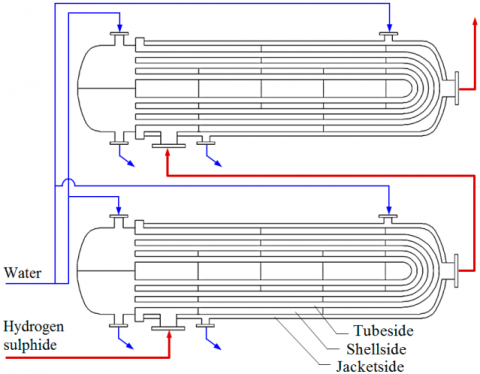Figure 1. Hydrogen sulphide gas coolers pair

Three experimental observations were performed for eight hours on alternate days, after starting the same heat exchangers pair in the cooling service. During each cycle, twenty measurements of the mass flowrates, inlet and outlet temperatures were recorded for every stream (tubeside water, hydrogen sulphide and jacketside water) on each cooler. A 120 rows database was arranged.

The following instrumentation was used:

•   Temperatures: industrial thermowells and Ashcroft bimetallic thermometers, 0.1 K measurement precision;

•   Water flowrate: Proline Prosonic Flow 93T portable flowmeter, 6.3·10-6 m3/s measurement precision;

•   Hydrogen sulphide flowrate: 4-20 mA process signal to a Siemens S7-400 PLC and Citect SCADA 7.10 system, 10-4 kg/s measurement precision.

3. ANN Model Development

3.1 Methodology overview

A multilayer perceptron (MLP) was used for prediction of the hydrogen sulphide gas coolers outputs. According to Mohanraj et al. (2015), this is the most suitable ANN architecture for heat exchangers modeling and simulation . Those operational parameters having a relevant effect on desired outputs were considered. Therefore, selected input variables were inlet temperatures, mass flowrates and time, while the response variables conformed to the outlet temperatures of involved three fluids (Figure 2). Operating time was included as an independent variable due to its influence on fouling factor and, consequently, deleterious effect on the overall heat transfer coefficient .

## f2.pngFigure 2. ANN schematic model

The number of neurons in the input and output layers equals the number of independent and dependent variables, respectively [3, 4, 6]. Optimization of the number of hidden layers and hidden neurons was performed by means of a network growing strategy, because smaller networks with fewer weights and biases usually generalize better. Besides, this is a more efficient approach than pruning algorithms, where the majority of the learning time is dedicated to configurations that are bigger than necessary .

A total of 27 network structures were tried and results subsequently compared. Training, validation and testing of every network configuration was carried out by using 120 data points, obtained through the experimental method. Levenberg-Marquardt (LM) and Bayesian Regularization (BR) back propagation learning algorithms were implemented during this stage (refer to section 3.2). Extrapolation capability was determined by comparing the trained neural network outputs against the predictions made by a phenomenological model, for a different set of 20 data points that was gathered by means of a central composite design (refer to section 3.3). The optimized ANN model was chosen on the basis of its predictive ability performance, by computing the correlation coefficient and the MAPE for the entire 140 points databases. MATLAB was used for all calculations.

3.2 Networks training, validation and testing

The ANN optimization was performed by changing the number of hidden neurons and hidden layers, as well as the training algorithm, to find out the network architecture with best predictive capability. The number of hidden layers was studied from 1 to 3, while total number of hidden neurons was varied from 1 to 19, with an increment of two (Table 1).

Table 1. Studied ANN structures

 Case Hidden layers Hidden neurons Network configuration 1 1 6-1-3 2 3 6-3-3 3 5 6-5-3 4 7 6-7-3 5 1 9 6-9-3 6 11 6-11-3 7 13 6-13-3 8 15 6-15-3 9 17 6-17-3 10 3 6-2-1-3 11 5 6-3-2-3 12 7 6-4-3-3 13 9 6-5-4-3 14 2 11 6-6-5-3 15 13 6-7-6-3 16 15 6-8-7-3 17 17 6-9-8-3 18 19 6-10-9-3 19 3 6-1-1-1-3 20 5 6-3-1-1-3 21 7 6-3-3-1-3 22 9 6-5-3-1-3 23 3 11 6-5-3-3-3 24 13 6-5-5-3-3 25 15 6-5-5-5-3 26 17 6-7-5-5-3 27 19 6-7-7-5-3

The log-sigmoid transfer function (logsig) was used for all hidden layers, thus allowing the network to learn linear and nonlinear relationships between input and output vectors. As logsig generates outputs between 0 and 1, it provides positive values for the response variables as expected for the investigated heat exchangers outlet temperatures. However, the linear transfer function (purelin) was employed for the output layers, to ensure that model responses are not limited to a small range of values as it happens if sigmoid neurons are utilized .

Every network structure was trained with two back propagation algorithms: LM and BR. The first learning procedure is a second-order numerical optimization technique that combines the advantages of Gauss-Newton and steepest descent algorithms. In general, for function approximation problems, it has higher stability and faster convergence for moderate size networks as compared to other training algorithms. The second one is an automated mathematical process that converts a nonlinear regression into a well-posed statistical problem, in a ridge regression manner. It minimizes a combination of squared errors and weights, and then determines the correct solution so as to produce a network that generalizes well. The BR training algorithm is considered as one of the best approaches to overcome the overfitting tendencies of neural networks, although it generally takes longer to converge than early stopping. In both cases the performance function was set to the MSE [10, 15-16].

Inputs and targets data were all normalized to fall into the range between 0 and 1, in order to make the training procedure more efficient and enhance the learning speed [3, 15-16]. Eq(1) was used for this purpose.

${{Y}_{i}}=\frac{{{X}_{i}}-\min (X)}{\max (X)-\min (X)}$   (1)

Where: Y- normalized value; X- primary data.

Since networks were trained under supervision, the experimental data was randomly divided into three data sets when applying the LM algorithm: 70 % for training, 15 % for validation and 15 % for testing. Each set was selected to be representative of all points in the entire experimental range [4, 10, 13]. In the case of the BR algorithm 30 % of the data was used for testing, as this procedure does not require that the validation data set be separate from the training one . MATLAB default training parameters were applied.

After completion of the training stage every network structure was tested and outlet temperatures prediction performances were compared. The configuration reporting higher overall correlation coefficient and lower MAPE was chosen as the best performing network.

3.3 Extrapolation capability assessment
3.3.1 Extrapolation test database

The 20 points database used to determine the networks extrapolation capability was analytically obtained by varying the fluids mass flowrates, which are the three independent variables prone to significant changes within the hydrogen sulphide cooling process. A central composite design was applied, consisting of a 23 full factorial design extended with properly planned extra runs (Figure 3). This approach is very useful for fitting response-surface models and optimization .

Points at the corners of the central composite cube were defined by experimental poles plus/minus the standard deviation, whereas the star points outside the face-center of the cube were calculated by adding/subtracting two times the standard deviation (Table 2). According to a standard normal distribution, 68.26 % and 95.44 % of the scores lie within one and two times the standard deviation, respectively .

The flowrate-related input variables were determined as per the above, although fixed values were considered for remaining independent variables. While means were used for inlet temperatures (307.45 K for water and 405.83 K for hydrogen sulphide), the maximum magnitude was considered for the operating time (8 h). On the other hand, values of the response variables were calculated through the model described on next section.

## f3.png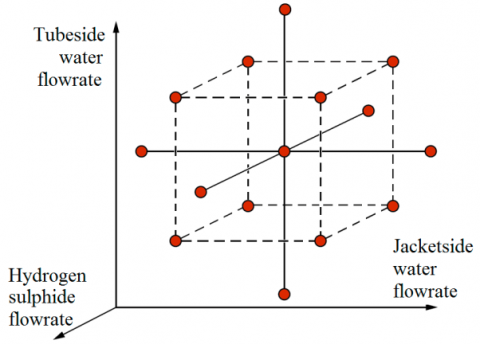Figure 3. Three-factors central composite design

Table 2. Extrapolation test database input variables

 Mass flowrates (kg/s) No. Tubeside water Hydrogen sulphide Jacketside water 1 0.5310 1.0301 0.2140 2 0.6390 1.0301 0.2140 3 0.5310 1.1515 0.2140 4 0.6390 1.1515 0.2140 5 0.5310 1.0301 0.2370 6 0.6390 1.0301 0.2370 7 0.5310 1.1515 0.2370 8 0.6390 1.1515 0.2370 9 0.5850 1.0908 0.2255 10 0.5850 1.0908 0.2255 11 0.5850 1.0908 0.2255 12 0.5850 1.0908 0.2255 13 0.5130 1.0908 0.2255 14 0.6570 1.0908 0.2255 15 0.5850 1.0027 0.2255 16 0.5850 1.1789 0.2255 17 0.5850 1.0908 0.2100 18 0.5850 1.0908 0.2410 19 0.5850 1.0908 0.2255 20 0.5850 1.0908 0.2255

3.3.2 Reference phenomenological model

A phenomenological model was established to compute the outlet temperatures of the three streams involved in the hydrogen sulphide cooling process, as function of mass flowrates, inlet temperatures and fluids thermo-physical properties. Basic relationships used for this purpose were: Eq(2) the heat transfer rate equation, based on first law of thermodynamics under no phase change and constant specific heats; Eq(3) the overall energy balance applied to the studied heat exchangers assuming steady-state, steady flow, plus no heat losses to the surroundings [2, 19].

$\forall n\in \{1,2,3\}$   ${{Q}_{n}}={{(\dot{m}\cdot Cp)}_{n}}\cdot \Delta {{T}_{n}}$ (2)

${{Q}_{2}}={{Q}_{1}}+{{Q}_{3}}$ (3)

where: Q – heat transfer rate, W; $\dot{m}$ – mass flowrate, kg/s; Cp – specific heat at constant pressure, J/(kg·K); $\Delta T$– temperature difference, K. Subscripts:

– first fluid (tubeside water); – second fluid (shellside hydrogen sulphide); – third fluid (jacketside water). Key properties of the main process stream are shown below (Table 3) .

Table 3. Hydrogen sulphide thermo-physical properties

 Property Description / Value Units Physical state Gas - Molecular weight 34.081 kg/kmol Density * 9.224 kg/m3 Dynamic viscosity * 1.59·10-5 Pa·s Specific heat * 1086.911 J/(kg·K) Thermal conductivity * 0.229 W/(m·K)

* Note: Fluid properties at 363.15 K and 0.79 MPa (absolute).

By rearranging Eq(2), both water streams outlet temperatures are calculated as:

$\forall n\in \{1,3\}\text{ and }\forall k\in \{a,b\}$     ${{T}_{n\text{ out}}}={{T}_{n\text{ in}}}+\frac{{{Q}_{k}}}{{{C}_{n}}}$ (4)

where: T – fluid temperature, K; C – heat capacity rate, W/K, used to replace the term $(\dot{m}\cdot Cp)$. Subscripts: a – inner thermal communication, between tubeside and shellside fluids; b – outer thermal communication, between shellside and jacketside fluids; in– inlet conditions; out– outlet conditions.

Once all water temperatures are known, the hydrogen sulphide exit temperature is determined by substituting Eq(2) into Eq(3) and rearranging terms:

${{T}_{2\text{ out}}}={{T}_{2\text{ in}}}-\frac{{{C}_{1}}({{T}_{1\text{ out}}}-{{T}_{1\text{ in}}})+{{C}_{3}}({{T}_{\text{3 out}}}-{{T}_{\text{3 in}}})}{{{C}_{2}}}$ (5)

Inner and outer heat transfer rates (${{Q}_{a}}$ and ${{Q}_{b}}$) required to compute the outlet temperatures according to Eq(4) were calculated through the Effectiveness–Number of Transfer Units (ε-NTU) method . In this context, governing equations are:

$\forall k\in \{a,b\}\text{ and }\forall n\in \{1,3\}$   ${{Q}_{k}}={{\varepsilon }_{k}}\cdot \min ({{C}_{n}},{{C}_{2}})\cdot ({{T}_{2\text{ in}}}-{{T}_{n\text{ in}}})$ (6)

$\forall k\in \{a,b\}$     ${{\varepsilon }_{k}}=f(NT{{U}_{k}},\text{ }C{{r}_{k}},\text{ flow arrangement})$ (7)

where: $\varepsilon$– exchanger heat transfer effectiveness; NTU – Number of Transfer Units; Cr – heat capacity rate ratio. The formulas for NTU and Cr are given by Eq(8) and Eq(9), respectively.

$\forall k\in \{a,b\}\text{ and }\forall n\in \{1,3\}$     $NT{{U}_{k}}={{A}_{k}}\cdot {{U}_{k}}/\min ({{C}_{n}},{{C}_{2}})$ (8)

$\forall k\in \{a,b\}\text{ and }\forall n\in \{1,3\}$    $C{{r}_{k}}=\min ({{C}_{n}},{{C}_{2}})/max({{C}_{n}},{{C}_{2}})$ (9)

where: A – heat transfer surface area, m2; U – overall heat transfer coefficient, W/(m2·K).

As shown in Eq(7), the heat transfer effectiveness can be expressed as function of the Number of Transfer Units, the heat capacity rate ratio and heat exchanger type. Hence, the inner thermal communication effectiveness, necessary to compute the heat transfer rate as per Eq(6), is based on

a shell-and-tube exchanger, TEMA “E” shell, mixed fluid configuration:

${{\varepsilon }_{a}}=2\cdot {{\left[ 1+C{{r}_{a}}+\sqrt{1+C{{r}_{a}}^{2}}\cdot \frac{1+\exp \left( -NT{{U}_{a}}\cdot \sqrt{1+C{{r}_{a}}^{2}} \right)}{1-\exp \left( -NT{{U}_{a}}\cdot \sqrt{1+C{{r}_{a}}^{2}} \right)} \right]}^{-1}}$ (10)

In an analogous manner, the outer thermal communication effectiveness is calculated by considering a counterflow heat exchanger configuration:

${{\varepsilon }_{b}}=\frac{1-\exp \left[ -NT{{U}_{b}}\cdot (1-C{{r}_{b}}) \right]}{1-C{{r}_{b}}\cdot \exp \left[ -NT{{U}_{b}}\cdot (1-C{{r}_{b}}) \right]}$     (11)

astly, the overall heat transfer coefficients are determined to compute the Number of Transfer Units ($NT{{U}_{a}}$ and $NT{{U}_{b}}$) according to Eq(8). Under fouled conditions, the inner thermal communication coefficient is calculated by Eq(12), while the outer one is given by Eq(13):

$U_{a}=\left[\frac{d_{o}}{d_{i} \cdot h_{1}}+\frac{d_{o} \cdot 1 n\left(d_{o} / d_{i}\right)}{2 \cdot k_{\text { tubes }}}+\frac{1}{h_{2}}+R_{f a}\right]^{-1}$ (12)

$U_{b}=\left[\frac{1}{h_{2}}+\frac{D_{i} \cdot 1 n\left(D_{o} / D_{i}\right)}{2 ? k_{s h e l l}}+\frac{D_{i}}{D_{o} \cdot h_{3}}+R_{f b}\right]^{-1}$ (13)

where: di and do – tubes inside and outside diameter, m; Diand Do – shell inside and outside diameter, m; h – convective heat transfer coefficient, W/(m2·K); ktubes and kshell – metal thermal conductivity, W/(m·K); Rf – fouling thermal resistance, m2·K/W.

The convective heat transfer coefficients were calculated as follows:

•   Tubeside fluid (h1): Nusselt number as per Seider & Tate correlations .

•   Shellside fluid (h2): Taborek method for shellside single-phase flow, shell-and-tube heat exchangers with single segmental baffles .

•   Jacketside fluid (h3): Nusselt number as per Ghiwala & Matawala correlation, valid for water laminar flow through annular sections .

Since the controlling resistance has a remarkable effect on the precision of proposed approach, fouling thermal resistances were estimated by means of a multivariable regression model :

\begin{align} {{R}_{fa}}=0,644582-0,558398\cdot {{{\dot{m}}}_{2}}+0,00337132\cdot t- & \\ -0,0000468447\cdot {{T}_{2\text{ in}}} & \\\end{align} (14)

\begin{align} {{R}_{fb}}=0,3923-0,176388\cdot {{{\dot{m}}}_{3}}-0,168991\cdot {{{\dot{m}}}_{2}}+ & \\ +0,00114202\cdot t-0,000510782\cdot {{T}_{3\text{ in}}}- & \\ -0,000013262\cdot {{T}_{2\text{ in}}} & \\\end{align} (15)

where: t– operating time, h.

Eq(14) and Eq(15) not only considers the fouling process dynamic nature, but also allows fouling factors to be determined from experience, with same-service heat exchangers. References on sulphur buildup fouling were not found elsewhere.

4. Results and Discussion

4.1 Phenomenological model validation

The proposed phenomenological model was validated by comparing predicted results against measured outlet temperatures, using the experimental data points (Figure 4).

Computed values of statistical criteria and error indexes confirmed the satisfactory agreement between predictions and observations (Table 4), thus considering this reference model appropriate for assessing the extrapolation capability of studied ANN configurations.

## f4-a.png(a) Tubeside cooling water

## f4-b.png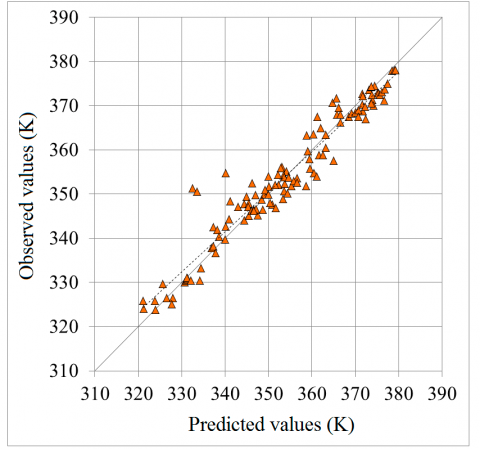(b) Shellside hydrogen sulphide gas

## f4-c.png(c) Jacketside cooling water

Figure 4. Phenomenological model verification

Table 4. Phenomenological model statistical results

 Outlet temperature prediction Criteria Tubeside water Hydrogen sulphide Jacketside water R2 0.8870 0.9339 0.9116 R 0.9418 0.9664 0.9548 MAPE 0.36 0.78 0.33 MAE 1.14 2.77 1.07
Where: R2 – coefficient of determination; R – correlation coefficient; MAPE – mean absolute percentage error, %; MAE – mean absolute error, K.
4.2 ANN structures and learning algorithms analysis
Correlations above 98.32 % and MAPE below 0.80 % were obtained during training, validation and testing of studied ANN structures by using the experimental data. Nevertheless, lower predictive ability performances were identified when assessing the extrapolation capability of each network configuration. For the unseen data set, correlations were found within the range from 91.74 % to 98.52 %, while MAPE varied from 0.91 % to 1.95 %. This last criterion had a significant influence on the overall correlation coefficient and overall MAPE, which resulted from networks performance evaluation over the entire 140 points database (Figures 5 to 7).

## f5-a.png(a) Correlation

## f5-b.png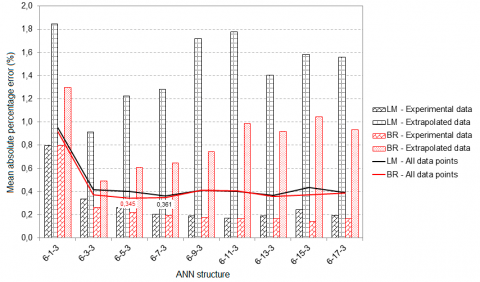(b) Mean relative error
Figure 5. Prediction performances, 1 hidden layer MLPs

## f6-a.png(a) Correlation

## f6-b.png(b) Mean relative error
Figure 6. Prediction performances, 2 hidden layers MLPs

## f7-a.png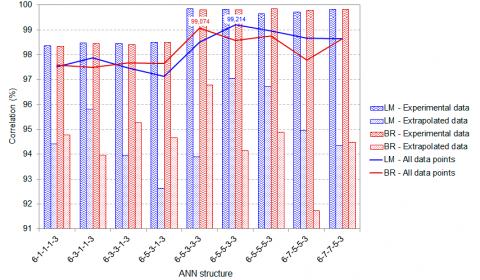(a) Correlation

## f7-b.png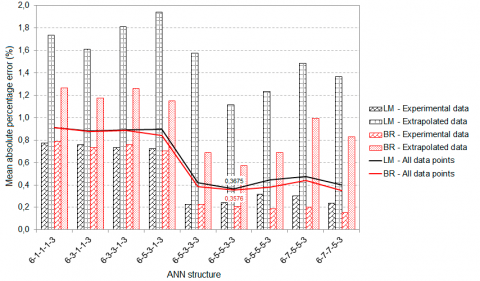(b) Mean relative error

Figure 7. Prediction performances, 3 hidden layers MLPs

Most of the ANN structures showed higher accuracy for prediction of hydrogen sulphide gas coolers outputs when trained with the LM algorithm, as compared to the BR method. It informs that, for improving generalization, the early stopping method implemented by MATLAB performed as good as the automated regularization.

Model performance was not only dependent on network configuration, as mostly expressed in consulted literature. Proper learning algorithm selection is also important for ANN optimization.

4.3 Proposed ANN model

The optimum ANN model for prediction of hydrogen sulphide gas coolers outlet temperatures was the 6-5-4-3 MLP, trained with the LM algorithm. This architecture provided an overall correlation of 99.47 % and a MAPE equal to 0.33 %. In terms of accuracy, it is comparable to other shell-and-tube heat exchanger ANN models (Table 5).

Table 5. Comparison with other studies MLPs

 Research Optimized structure Achieved correlation Pandharipande et al.  4-15-15-15-2 95.0-98.0 % Mandavgane & Pandharipande  4-15-15-15-2 98.0-99.5 % Xie et al.  8-6-5-3 92.0 % Moghadassi et al.  Several 96.0-100 % Jasim  3-16-16-16-2 100 % Iyengar  4-6-3 98.0-99.0 % This research 6-5-4-3 99.47 %

Computed weights and biases are shown below (Figure 8).

The predictive ability performance was also corroborated by plotting the network outputs against expected exit temperatures, using the full data set (Figure 9). Even when a higher correlation was obtained for the hydrogen sulphide temperature, larger deviations were found on predictions related to this stream. The average absolute error was 0.98 K as compared to the experimental readings, while equal to 6.03 K if assessed versus the phenomenological model outputs. These differences have no significant implication over the hydrogen sulphide cooling process, therefore are acceptable for this system study.

## f8.png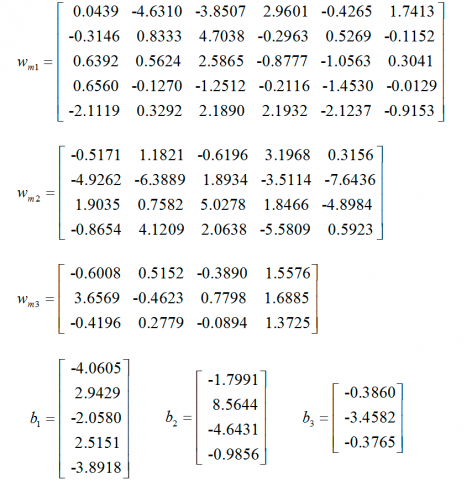Figure 8. 6-5-4-3 perceptron weights and biases values

## f9-a.png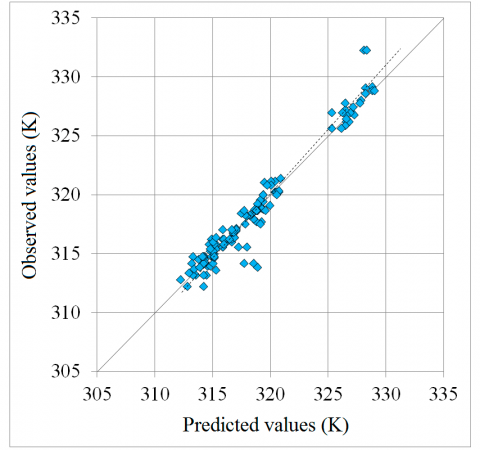(a) Tubeside cooling water

## f9-b.png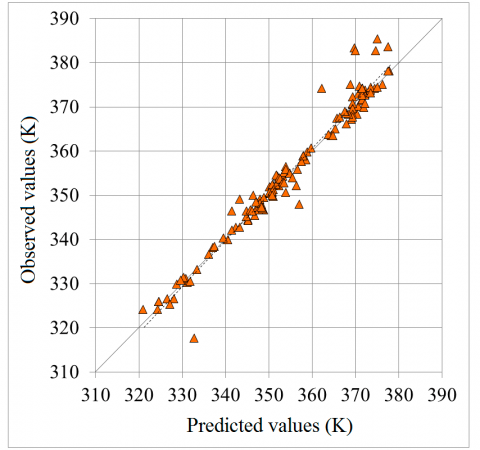(b) Shellside hydrogen sulphide gas

## f9-c.png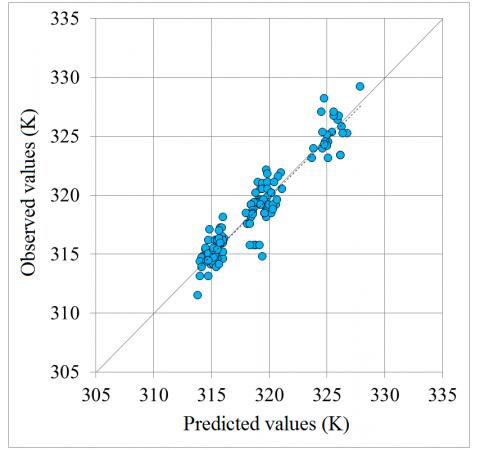(c) Jacketside cooling water

Figure 9. ANN model verification

Despite proposed perceptron was found to be effective for extrapolation beyond the training data, within the conditions evaluated on this research, some model responses are deprived of physical meaning. Heat transfer rate unbalances ${{Q}_{2}}-({{Q}_{1}}+{{Q}_{3}})\ne 0$ were noticed when using the extrapolation test database for simulation of the heat exchangers, as well as non-controlling streams having a major effect on the hydrogen sulphide gas outlet temperature (Figure 10).

According to the ANN model outputs, released heat markedly differs from absorbed heat on data points 3, 4, 7 and 8, where the hydrogen sulphide gas flowrate is evaluated at its next to highest value (refer to Table 2 on section 3.3.1). A similar behavior was exposed for data points between 14 and 18, where the extreme flowrates of each stream were the inputs. On the other side, it can be seen that the effect of the tubeside water flowrate over the hydrogen sulphide outlet temperature is sometimes overrated by the ANN model. As cooling water is not the lowest convective heat transfer coefficient stream, for constant hydrogen sulphide flowrates (actually the controlling fluid) the difference between predicted outlet temperatures should be minimum. The overrate is confirmed by comparing the perceptron responses against the phenomenological model outputs for data point pairs 3-4, 7-8, as well as 13-14.

## f10.pngFigure 10. Model responses analysis

5. Conclusions

An ANN model has been proposed after a network structure and learning algorithm rigorous selection. In this respect, the 6-5-4-3 MLP trained with the LM method is recommended for prediction of hydrogen sulphide gas coolers outlet temperatures. It attained a 99.47 % overall correlation and 0.33 % MAPE. Extrapolation capability was confirmed by computing same performance parameters for data points beyond the training set, in this manner obtaining a correlation of 98.52 % and a MAPE equal to 0.97 %.

Proposed model will not only facilitate the study of different plant operational scenarios, but also doing the analysis without the risk of exposures to fugitive emissions of a lethal compound like hydrogen sulphide. However, it is not recommended to employ the neural network outputs for physical understanding of heat transfer processes occurring within studied heat exchangers.

Nomenclature
References

 Chou S, Ogden JM, Pohl HR, Scinicariello F, Ingerman L, Barber L, Citra M. (2016). Toxicological Profile for Hydrogen Sulphide and Carbonyl Sulphide. U.S. Department of Health & Human Services, Agency for Toxic Substances and Disease Registry. Atlanta, USA.

 Shah RK, Sekulić DP. (2003). Fundamentals of Heat Exchangers Design. John Wiley & Sons Inc., New Jersey, USA.

 Kamble LV, Pangavhane DR, Singh TP. (2014). Heat transfer studies using artificial neural network - a review. International Energy Journal 14: 25-42.

 Mohanraj M, Jayaraj S, Muraleedharan C. (2015). Applications of artificial neural networks for thermal analysis of heat exchangers – a review. International Journal of Thermal Sciences 90: 150-172. https://doi.org/10.1016/j.ijthermalsci.2014.11.030

 Romero-Méndez R, Lara-Vázquez P, Oviedo-Tolentino F, Durán-García HM, Pérez-Gutiérrez FG, Pacheco-Vega A. (2016). Use of artificial neural networks for prediction of the convective heat transfer coefficient in evaporative mini-tubes. Ingeniería Investigación y Tecnología XVII (1): 23-34. https://doi.org/10.1016/j.riit.2016.01.003

 Hakeem MA, Kamil M. (2017). Studies on artificial neural network based prediction of wall temperature profiles for methanol-water system in a natural circulation thermosiphon reboiler. Heat and Mass Transfer Research Journal 1(1): 61-78.

 Pandharipande SL, Siddiqui MA, Dubey A, Mandavgane SA. (2004). Optimizing ANN architecture for shell and tube heat exchanger modelling. Indian Journal of Chemical Technology 11: 804-810.

 Mandavgane SA, Pandharipande SL. (2006). Application of optimum ANN architecture for heat exchanger modelling. Indian Journal of Chemical Technology 13: 634-639.

 Xie GN, Wang QW, Zeng M, Luo LQ. (2007). Heat transfer analysis for shell and tube heat exchangers with experimental data by artificial neural networks approach. Applied Thermal Engineering 27(5): 1096-1104. https://doi.org/10.1016/j.applthermaleng.2006.07.036

 Moghadassi AR, Hosseini SM, Parvizian F, Mohamadiyon F, Behzadi-Moghadam A, Sanaeirad A. (2011). An expert model for the shell and tube heat exchangers analysis by artificial neural networks. ARPN Journal of Engineering and Applied Sciences 6(9): 78-93.

 Jasim HH. (2013). Estimated outlet temperatures in shell-and-tube heat exchanger using artificial neural network approach based on practical data. Al-Khwarizmi Engineering Journal 9(2): 12-20.

 Iyengar AS. (2015). Thermal analysis of shell and tube heat exchangers using artificial neural networks. Ethiopian Journal of Science and Technology 8(2): 107-120. https://doi.org/10.4314/ejst.v8i2.5

 Harrison J (editor). (2007). Standards of the Tubular Exchanger Manufacturers Association, 9 ed. TEMA Inc., New York, USA.

 Naphon P, Arisariyawong T, Nualboonrueng T. (2016). Artificial neural network analysis on the heat transfer and friction factor of the double tube with spring insert. International Journal of Applied Engineering Research 11(5): 3542-3549.

 Beale MH, Hagan MT, Demuth HB. (2016). Neural Network Toolbox™ User’s Guide. The MathWorks Inc., Massachusetts, USA.

 Ghritlahre HK, Prasad RK. (2018). Investigation on heat transfer characteristics of roughened solar air heater using ANN technique. International Journal of Heat and Technology 36(1): 102-110. https://doi.org/10.18280/ijht.360114

 Kleppmann W, Aalen H. (2014). Design of experiments (DoE): Optimizing Products and processes efficiently. Chemical Engineering 121(11): 50-57.

 Moore DS, Notz WI, Fligner MA. (2015). The Basic Practice of Statistics, 7 ed. W.H. Freeman and Company, New York, USA.

 Ghiwala TM, Matawala VK. (2014). Sizing of triple concentric pipe heat exchanger. International Journal of Engineering Development and Research 2(2): 1683-1692.

 Seider EN, Tate GE. (1936). Heat transfer and pressure drop of liquids in tubes. Industrial and Engineering Chemistry 28(12): 1429–1435. https://doi.org/10.1021/ie50324a027

 Taborek J. (1983) Shell-and-tube heat exchangers: Single phase flow. In: Schlünder EU (editor-in-chief). Heat Exchanger Design Handbook. Hemisphere Publishing Corporation, New York, USA.

 Sánchez-Escalona AA, Góngora-Leyva E, Zalazar-Oliva C. (2018). Prediction of the fouling thermal resistance on the hydrogen sulphide gas coolers (in Spanish). Minería y Geología 34(3): 345-359.